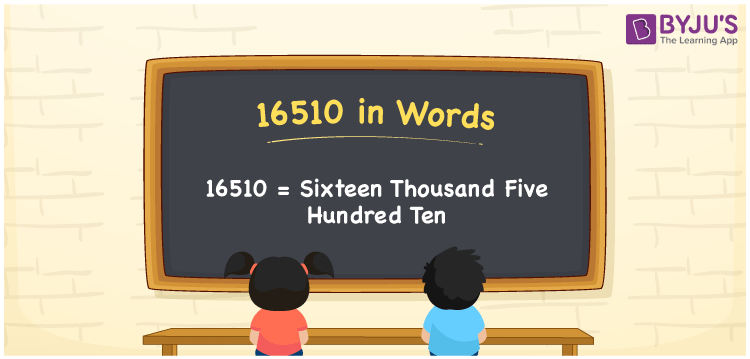# 16510 in Words

16510 in words is written as Sixteen Thousand Five Hundred Ten. For instance, Rashmi purchased a three-door wardrobe worth Rs. 16510, then you can say, “Rashmi purchased a three-door wardrobe worth Rupees Sixteen Thousand Five Hundred Ten”. We can convert the number 16510 quickly using a place value table. The number 16510 is a cardinal number as it represents a definite value. Let’s learn how to write the number 16510 in word form using a place value chart in this article.

 16510 in Words Sixteen Thousand Five Hundred Ten Sixteen Thousand Five Hundred Ten in numerical form 16510

## 16510 in English Words

Generally, using the letters of the English alphabet we write the numbers in words. Therefore, the number 16510 in English words is written as Sixteen Thousand Five Hundred Ten.## How to Write 16510 in Words?

16510 is a five-digit number. Hence, let us create a five-column table to convert the number 16510 into words. The place value chart for the number 16510 is given below.

 Ten Thousands Thousands Hundreds Tens Ones 1 6 5 1 0

Hence, we can write the expanded form as:

1 x Ten Thousand + 6 x Thousands + 5 x Hundred + 1 x Ten + 0 x One

= 1 x 10000 + 6 x 1000 + 5 x 100 + 1 x 10 + 0 x 1

= 10000 + 6000 + 500 + 10 + 0

= 10000 + 6000 + 500 + 10

= 16510

= Sixteen Thousand Five Hundred Ten

Therefore, 16510 in words is written as Sixteen Thousand Five Hundred Ten

Interesting way of writing 16510 in words

1 = One

16 = Sixteen

165 = One Hundred and Sixty-Five

1651 = One Thousand Six Hundred Fifty-One

16510 = Sixteen Thousand Five Hundred Ten

Thus, the word form of the number 16510 is Sixteen Thousand Five Hundred Ten

16510 is a natural number that is the successor of 16509 and the predecessor of 16511

• 16510 in words – Sixteen Thousand Five Hundred Ten
• Is 16510 an odd number? – No
• Is 16510 an even number? – Yes
• Is 16510 a perfect square number? – No
• Is 16510 a perfect cube number? – No
• Is 16510 a prime number? – No
• Is 16510 a composite number? – Yes

## Frequently Asked Questions on 16510 in Words

### Write 16510 in words.

16510 in words is Sixteen Thousand Five Hundred Ten.

### What is the value of 15000 + 1510 in words?

15000 + 1510 = 16510 The value of 15000 + 1510 is 16510. Hence, 16510 in words is Sixteen Thousand Five Hundred Ten.

### Write Sixteen Thousand Five Hundred Ten in numbers.

Sixteen Thousand Five Hundred Ten in numbers is 16510.